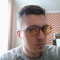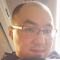Class Central is learner-supported. When you buy through links on our site, we may earn an affiliate commission.

Bayesian Statistics: From Concept to Data Analysis

Overview

Class Central Tips
This course introduces the Bayesian approach to statistics, starting with the concept of probability and moving to the analysis of data. We will learn about the philosophy of the Bayesian approach as well as how to implement it for common types of data. We will compare the Bayesian approach to the more commonly-taught Frequentist approach, and see some of the benefits of the Bayesian approach. In particular, the Bayesian approach allows for better accounting of uncertainty, results that have more intuitive and interpretable meaning, and more explicit statements of assumptions. This course combines lecture videos, computer demonstrations, readings, exercises, and discussion boards to create an active learning experience. For computing, you have the choice of using Microsoft Excel or the open-source, freely available statistical package R, with equivalent content for both options. The lectures provide some of the basic mathematical development as well as explanations of philosophy and interpretation. Completion of this course will give you an understanding of the concepts of the Bayesian approach, understanding the key differences between Bayesian and Frequentist approaches, and the ability to do basic data analyses.

Syllabus

• Probability and Bayes' Theorem
• In this module, we review the basics of probability and Bayes’ theorem. In Lesson 1, we introduce the different paradigms or definitions of probability and discuss why probability provides a coherent framework for dealing with uncertainty. In Lesson 2, we review the rules of conditional probability and introduce Bayes’ theorem. Lesson 3 reviews common probability distributions for discrete and continuous random variables.
• Statistical Inference
• This module introduces concepts of statistical inference from both frequentist and Bayesian perspectives. Lesson 4 takes the frequentist view, demonstrating maximum likelihood estimation and confidence intervals for binomial data. Lesson 5 introduces the fundamentals of Bayesian inference. Beginning with a binomial likelihood and prior probabilities for simple hypotheses, you will learn how to use Bayes’ theorem to update the prior with data to obtain posterior probabilities. This framework is extended with the continuous version of Bayes theorem to estimate continuous model parameters, and calculate posterior probabilities and credible intervals.
• Priors and Models for Discrete Data
• In this module, you will learn methods for selecting prior distributions and building models for discrete data. Lesson 6 introduces prior selection and predictive distributions as a means of evaluating priors. Lesson 7 demonstrates Bayesian analysis of Bernoulli data and introduces the computationally convenient concept of conjugate priors. Lesson 8 builds a conjugate model for Poisson data and discusses strategies for selection of prior hyperparameters.
• Models for Continuous Data
• This module covers conjugate and objective Bayesian analysis for continuous data. Lesson 9 presents the conjugate model for exponentially distributed data. Lesson 10 discusses models for normally distributed data, which play a central role in statistics. In Lesson 11, we return to prior selection and discuss ‘objective’ or ‘non-informative’ priors. Lesson 12 presents Bayesian linear regression with non-informative priors, which yield results comparable to those of classical regression.

Herbert Lee

Reviews

3.5 rating, based on 10 reviews

Start your review of Bayesian Statistics: From Concept to Data Analysis

•Grigory Shepelev
5

Grigory Shepelev is taking this course right now.

I am an average guy with average IQ and a little bit greater than average math abilities. I set up a goal for myself to learn statistics on a high level because I need it for my future career and applied math is something I am fascinated about. I do...
• Jon Ingram
15

Jon Ingram completed this course, spending 8 hours a week on it and found the course difficulty to be medium.

An interesting introduction to Bayesian statistics and inference. Not for people with no statistical background, as it does assume you are comfortable with various distributions, expectations, variances, etc. and the 'standard' frequentist worldview (including inferential procedures such as linear regression). The material was well explained, and generally well examined, with a mixture of multiple choice understanding questions, and numeric response tasks which also serve as a very basic introduction to R (or Excel if you are crazy). It was good to see the instructor realising that a light shirt was causing problems and switching to a darker one as the videos went on!
• Anonymous

Anonymous is taking this course right now.

I wanted to love the course, but as I go through it, the explanation became increasing scarce and it became really hard to follow. The video lecture became increasingly about writing formulas and iterating through them, rather than understanding what's really going on behind this. It assumes you have a lot of prior knowledge in Stats and remember them (I did, but it was a while a go and I need to be reminded).
•PJ Giacomo
1

PJ Giacomo is taking this course right now and found the course difficulty to be medium.

No doubt that the course is well structured
However, Dr Lee does not give enough explanation in the lectures. He just keeps writing formulas with very little clarification which makes it very challenging to grasp new concepts at such rushed paces.
If Bayesian statistics is new to you granted you need other resources to help you in this course
Very disappointing
• Jonathan Bechtel
4

Jonathan Bechtel completed this course.

I wanted to love this class, but I thought it was just okay. The material is important, but the delivery of the lessons always felt rushed, robbing the course of its potential impact. Dr. Lee has a tendency to speed through his explanations without...
• Shubham Agarwal completed this course, spending 4 hours a week on it and found the course difficulty to be medium.

I strongly recommend this course to those who are interested in learning theoretical concepts that build Machine Learning statistics especially Bayesian. The course content was well organized and the professor presented the concepts in a very engaging way. Relevant and appropriate examples and in-video quizzes were very helpful in understanding the theory.
• Anonymous

Anonymous completed this course.

A really great instructor. The subject is hard but the course is well structured and clearly explained. My only advice is to revise the coding component (some Python lab would be great)
• Bishal Lakha completed this course.

• Poppo Parin completed this course.

• Macemers
1

Macemers completed this course.Never Stop Learning!

Get personalized course recommendations, track subjects and courses with reminders, and more.